Learn All Concepts of Chapter 2 Class 11 Relations and Function - FREE. Check - Relation and Function Class 11 - All Concepts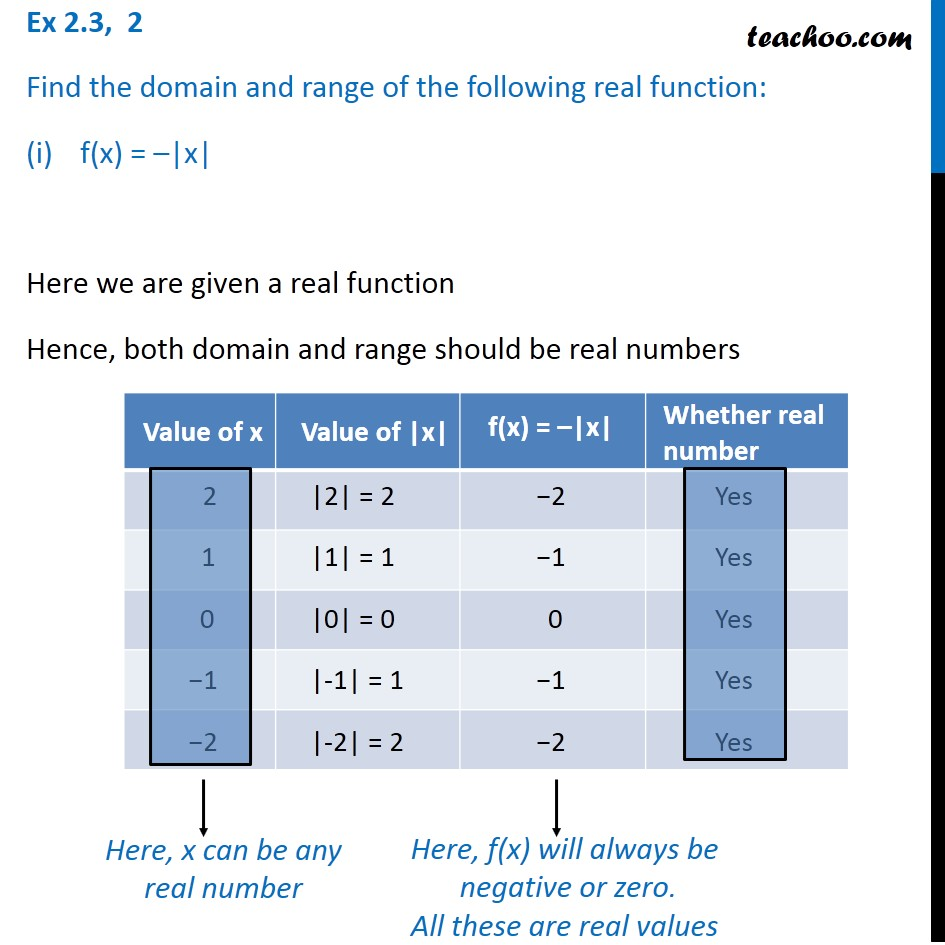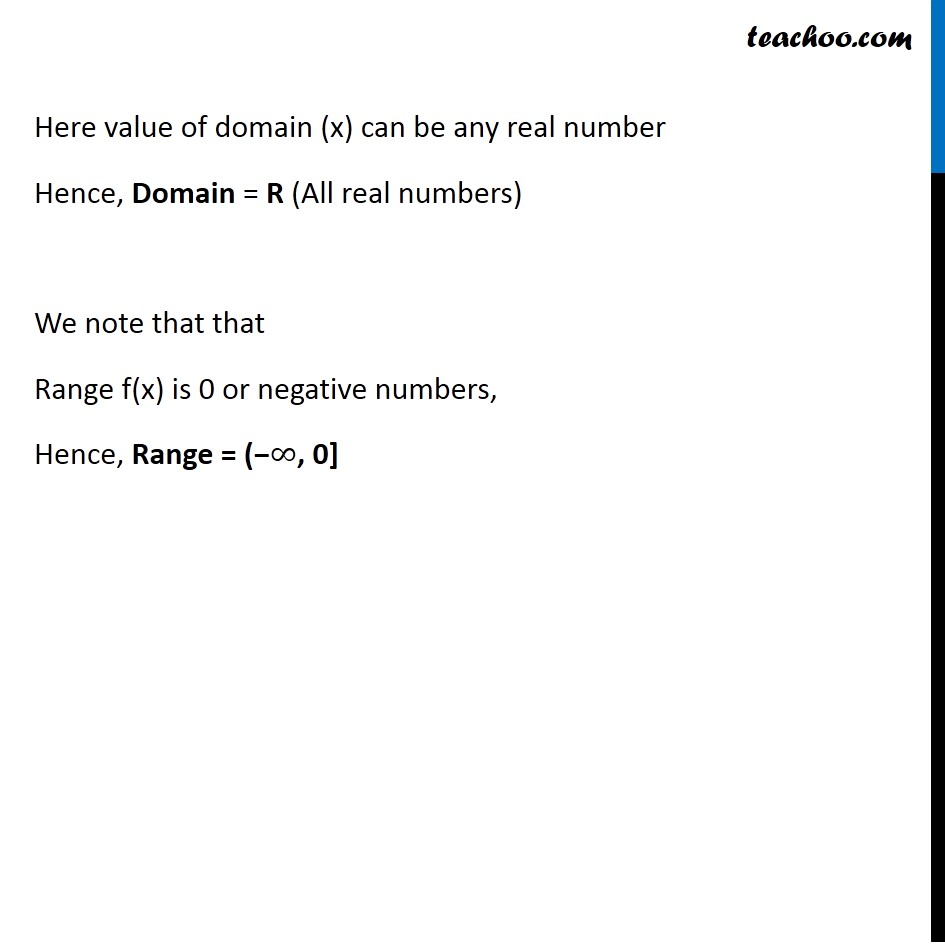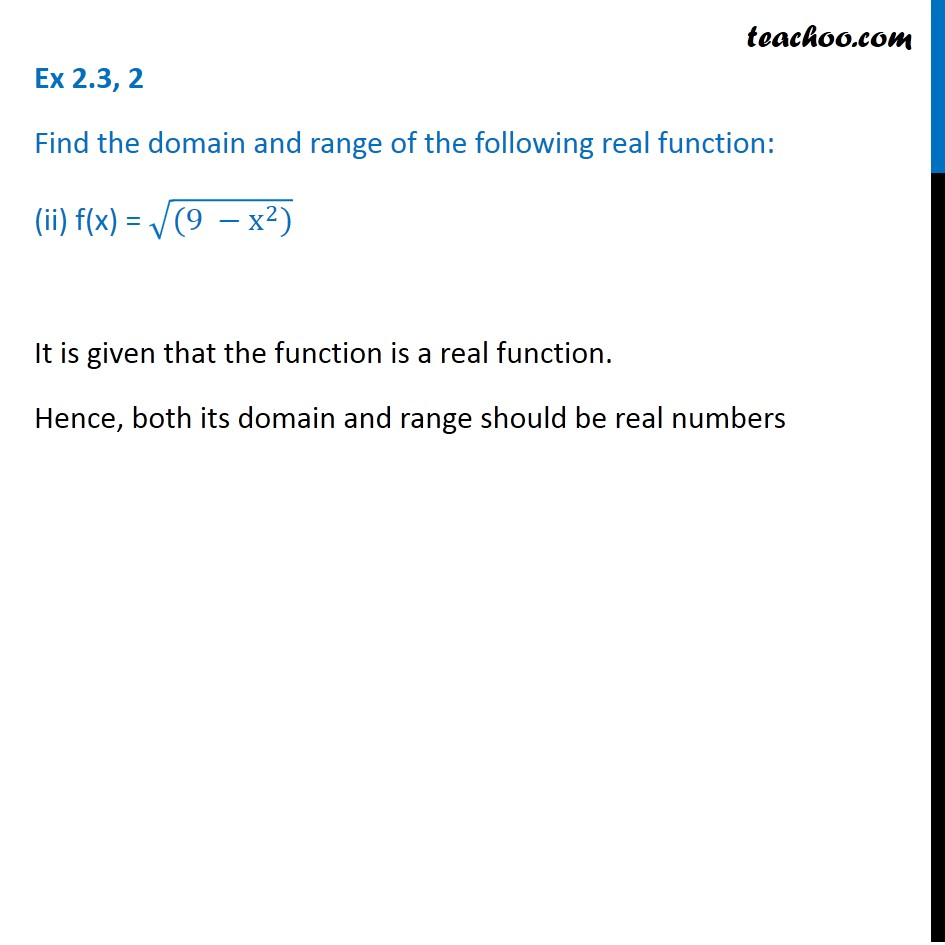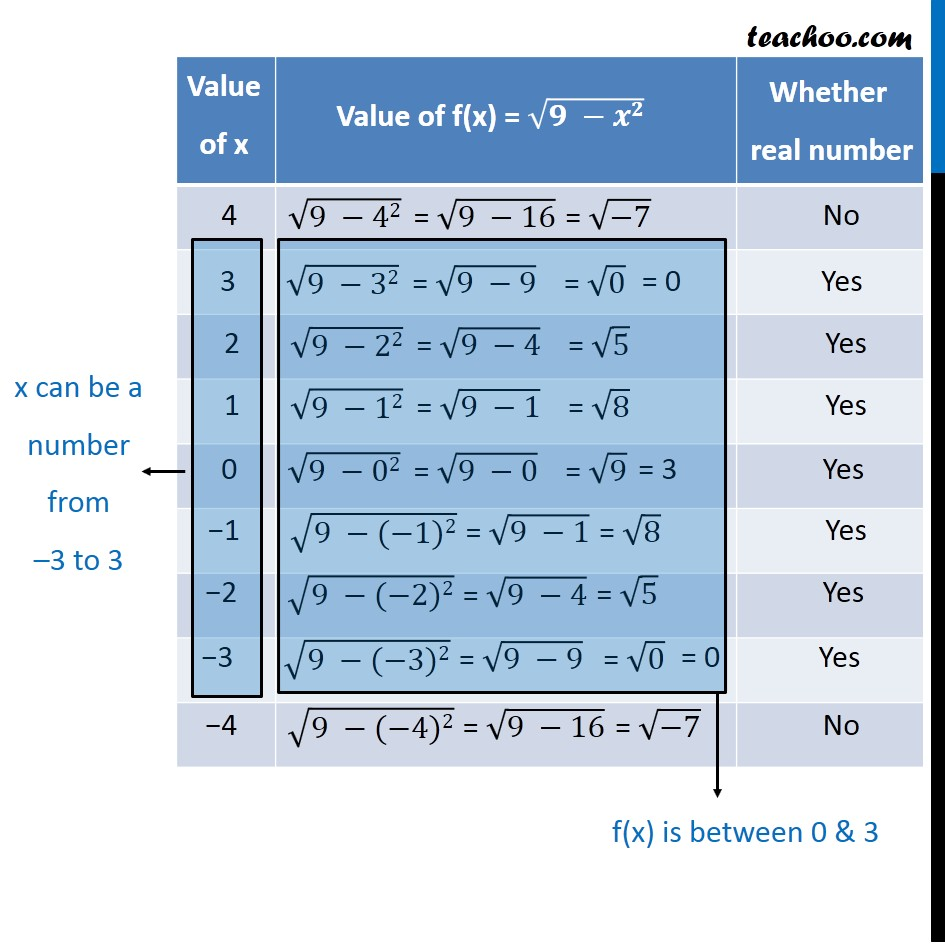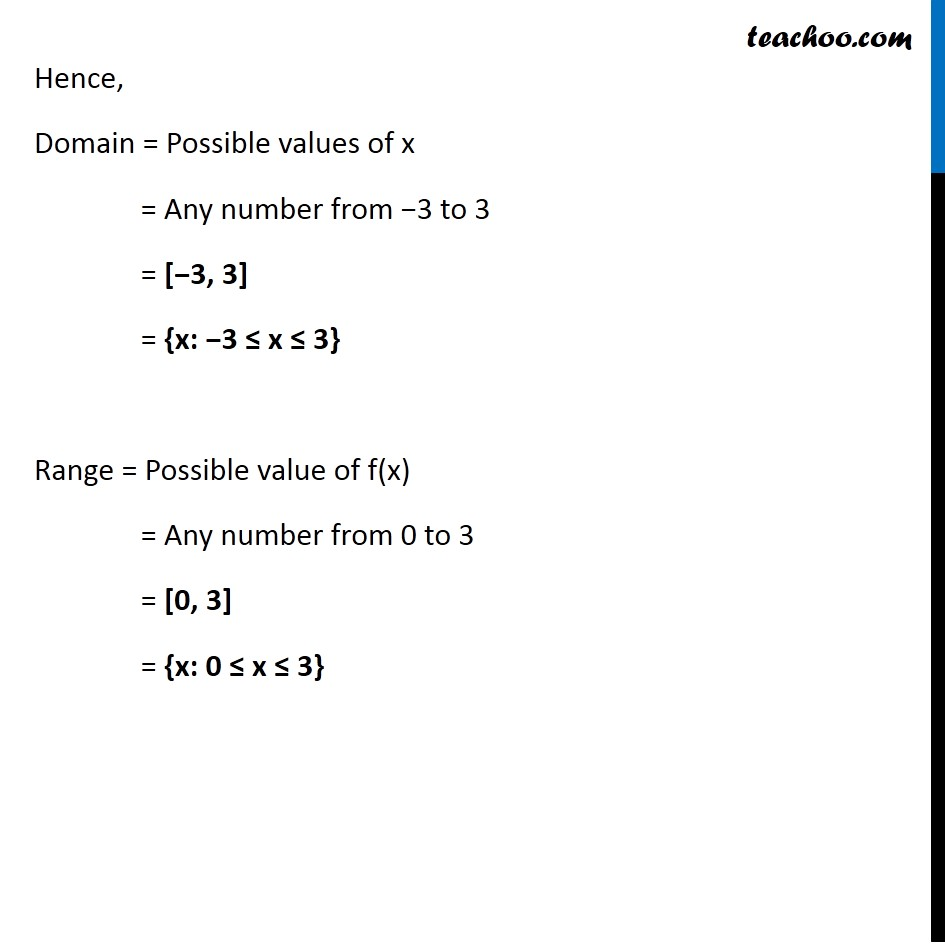1. Chapter 2 Class 11 Relations and Functions
2. Serial order wise
3. Ex 2.3

Transcript

Ex 2.3, 2 Find the domain and range of the following real function: f(x) = –|x| Here we are given a real function Hence, both domain and range should be real numbers Here, x can be any real number Here, f(x) will always be negative or zero. All these are real values Here value of domain (x) can be any real number Hence, Domain = R (All real numbers) We note that that Range f(x) is 0 or negative numbers, Hence, Range = (−∞, 0] Ex 2.3, 2 Find the domain and range of the following real function: (ii) f(x) = √((9 −x^2)) It is given that the function is a real function. Hence, both its domain and range should be real numbers x can be a number from –3 to 3 f(x) is between 0 & 3 Hence, Domain = Possible values of x = Any number from −3 to 3 = [−3, 3] = {x: −3 ≤ x ≤ 3} Range = Possible value of f(x) = Any number from 0 to 3 = [0, 3] = {x: 0 ≤ x ≤ 3}

Ex 2.3